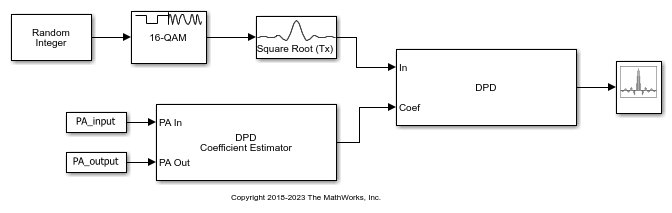Predistort Power Amplifier Input Signal in Simulink

Apply digital predistortion (DPD) to a 16-QAM signal of random symbols. The DPD Coefficient Estimator block uses a captured signal containing from input and output signals from a power amplifier (PA) to determine the predistortion coefficient matrix. Digital predistortion of the signal preconditions is to correct impairments that the PA introduces. This model does not include a block representing the PA.

The PreLoadFcn callback (found in File>Model Properties>Callbacks) initializes model parameters, and also loads workspace variables PA_Input and PA_Output from the file commpowamp_dpd_data.mat. The PA_Input and PA_Output variables are baseband-equivalent signals captured at the input and output of a PA. PA_Input and PA_Output are used by the DPD Coefficient Estimator block to estimate the memory-polynomial coefficients. The memory-polynomial coefficients are input to the DPD block to predistort the PA input signal.The input signal path of the model generates a random symbol stream, applies 16-QAM modulation, and then applies raised-cosine transmit filtering to the modulated signal.

The input signal is digitally predistorted in the DPD block using the memory-polynomial coefficients generated in the DPD Coefficient Estimator block. The DPD block returns the predistorted input signal for the PA that produced the distorted PA output signal.